# Independent functions, system of

A sequence of measurable functionssuch thatfor anyand any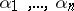. The simplest example of a system of independent functions is the Rademacher system.

(Kolmogorov's) criterion for the almost-everywhere convergence of a series of independent functions: For a series of independent functions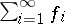to converge almost everywhere it is necessary and sufficient that for some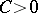the following three series converge: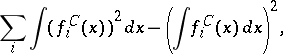whereOf course, to be able to introduce the concept of a system of independent functions one needs to have a measure space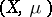on which the functions are defined and measurable (with respect to). Moreover,must be positive and finite, socan be taken a probability measure (thenis a probability space). An example is.
The notion of a system of independent functions (random variables) should not be mixed up with that of an independent set of elements of a vector spaceover a field: A set of elements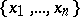insuch that for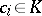,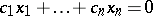implies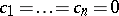, see also Vector space.